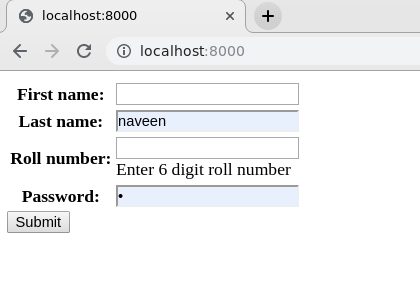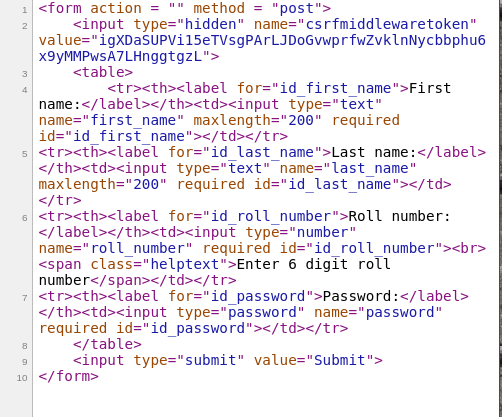Open In App

# {{ form.as_table }} – Render Django Forms as table

Django forms are an advanced set of HTML forms that can be created using python and support all features of HTML forms in a pythonic way. Rendering Django Forms in the template may seem messy at times but with proper knowledge of Django Forms and attributes of fields, one can easily create excellent Form with all powerful features. In this article, Form is rendered as table in the template.

#### {{ form.as_table }} – Render Django Forms as Table

Illustration of {{ form.as_table }} using an Example. Consider a project named geeksforgeeks having an app named geeks.

Refer to the following articles to check how to create a project and an app in Django.

Let’s create a sample Django Form to render it and show as an example. In geeks > forms.py, enter following code

## Python3

 `from` `django ``import` `forms`` ` `# creating a form``class` `InputForm(forms.Form):`` ` `    ``first_name ``=` `forms.CharField(max_length ``=` `200``)``    ``last_name ``=` `forms.CharField(max_length ``=` `200``)``    ``roll_number ``=` `forms.IntegerField(``                     ``help_text ``=` `"Enter ``6` `digit roll number"``                     ``)``    ``password ``=` `forms.CharField(widget ``=` `forms.PasswordInput())`

Now we need a View to render this form into a template. Let’s create a view,

## Python3

 `from` `django.shortcuts ``import` `render``from` `.forms ``import` `InputForm`` ` `# Create your views here.``def` `home_view(request):``    ``context ``=``{}``    ``context[``'form'``]``=` `InputForm()``    ``return` `render(request, "home.html", context)`

Finally, we will create the template where we need the form to be placed. In templates > home.html,

## html

 `<``form` `action = "" method = "post">``    ``{% csrf_token %}``    ``<``table``>``        ``{{ form.as_table }}``    ````    ``<``input` `type="submit" value="Submit">```

Here {{ form.as_table }} will render them as table cells wrapped in <tr> tags. Let’s check whether this is working accordingly or not. Open http://localhost:8000/Let’s check the source code whether the form is rendered as a table or not. By rendering as a table it is meant that all input fields will be enclosed in <tr> tags. Here is the demonstration,